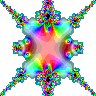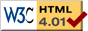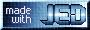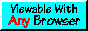# Complex Domain Coloring using fztopng

fztopng is a S-Lang script that converts a function of a complex number over a region of the complex plane into a PNG image. The script requires at least version 2.1.0 of the S-Lang library, as well as PNG support.

Running the script without any arguments will produce a usage message:

Version 0.1.0 Usage: ./fztopng [options] -f func | func.sl
Options:
-x|--real xlo:xhi:#N        Grid for x=Real(z) (default: -2:2:#512)
-y|--imag ylo:yhi:#N        Grid for y=Imag(z) (default: -2:2:#512)
--iter N                    Iterate N type (default=1)
--hsv                       Use HSV method (default)
--hue=float,float           Min/Max values of Hue ([0->360])
--sat=float,float           Min/Max values of Saturation ([0->1])
--val=float,float           Min/Max values of Value ([0->1])
--rgb=int,int               Min/Max RGB values for gradient method
-o file.png                 Output file (default: func.png)
-h|--help                   This message

Note: The file func.sl must contain a function called f_of_z.
If the -f expr form is used, it must involve z, e.g., -f sin(z)


As the usage message shows, the script implements two methods for associating a color with a complex number: the HSV method and the Gradient method. The HSV method maps the phase and modulus of the complex number into the color's HSV representation using:The above equations indicate that S and V lie in the range 0 to 1, whereas H is an angle that runs between 0 and 360 degrees. The --hue, --sat, and --val options may be used to further restrict the corresponding quantities to specified ranges. For example,

   fztopng --hue=240,270 myfunc.sl

would produce a "blueish" image with the hue lying between 240 and 270 degrees.

In contrast the Gradient method uses the phase of the complex number to linearly interpolate between two colors in the RGB representation. It does not use the modulus at all. The two RGB values may be specified using the --rgb option as integers expressed in the hexadecimal form 0xRRGGBB, e.g.,

   fztopng --grad --rgb=0xFF0000,0x00FFFF myfunc.sl

In this example, as the hue increases from 0 to 360 degrees, the red component decreases from 255 (0xFF) to 0, while at the same time the green and blue components increase from 0 to 255 (0xFF).

The --iter option specifies the number of times the function is to be iterated upon itself.

Unless specified by the -o option, the output PNG file will have the same name as the input function file except the .png filename extension will be used. For example,

   fztopng myfunc.sl

will produce a PNG file called myfunc.png.

There are two ways of specifying the function: via a file containing a S-Lang function called f_of_z, or via an expression on the command-line using the -f option. An example of the latter form is:

   fztopng -x -8:8:#512 -y -2:2:#128 -o sin_hsv.png -f "sin(z)"

This produces a PNG file called sin_hsv.png containing the 512 by 128 pixel image:The most flexibility is achieved using a file coded in S-Lang containing the function called f_of_z. See the gallery for some examples of this approach. Also the file could define a function f_of_z that reads a pre-computed complex array from the disk and returns it. This could be especially useful when dealing with very CPU intensive functions.

This page was last updated Nov 26, 2017 by John E. Davis. To comment on it or the material presented here, send email to jed at jedsoft org.